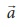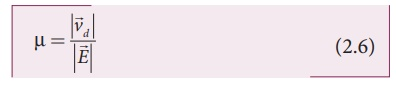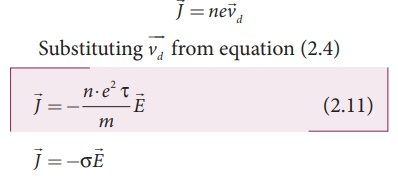Home | | Physics 12th Std | Electric Current

# Electric Current

When a potential difference is applied by the battery across the ends of the conductor, the free electrons drift towards the positive terminal of the battery, producing a net electric current.

ELECTRIC CURRENT

Matter is made up of atoms. Each atom consists of a positively charged nucleus with negatively charged electrons moving around the nucleus. Atoms in metals have one or more electrons which are loosely bound to the nucleus. These electrons are called free electrons and can be easily detached from the atoms. The substances which have an abundance of these free electrons are called conductors. These free electrons move at random throughout the conductor at a given temperature. In general due to this random motion, there is no net transfer of charges from one end of the conductor to other end and hence no current.

When a potential difference is applied by the battery across the ends of the conductor, the free electrons drift towards the positive terminal of the battery, producing a net electric current. This is easily understandable from the analogy given in the Figure 2.1.In the XI Volume 2, unit 6, we studied, that the mass move from higher gravitational potential to lower gravitational potential. Likewise, positive charge flows from higher electric potential to lower electric potential and negative charge flows from lower electric potential to higher electric potential. So battery or electric cell simply creates potential difference across the conductor.

The electric current in a conductor is defined as the rate of flow of charges through a given cross-sectional area A. It is shown in the Figure 2.2.If a net charge Q passes through any cross section of a conductor in time t, then the current is defined as I = Q/t . But charge flow is not always constant. Hence current can more generally be defined asWhere ÔłćQ is the amount of charge that passes through the conductor at any cross section during the time interval Ôłćt. If the rate at which charge flows changes in time, the current also changes. The instantaneous current I is defined as the limit of the average current, as  ╬ö t Ôćĺ 0The SI unit of current is the ampere (A)

That is, 1A of current is equivalent to 1 Coulomb of charge passing through a perpendicular cross section in 1second. The electric current is a scalar quantity.

### EXAMPLE 2.1

Compute the current in the wire if a charge of 120 C is flowing through a copper wire in 1 minute.

Solution

The current (rate of flow of charge) in the wire is

I = Q/t = 120/60 = 2A

## 1. Conventional CurrentIn an electric circuit, arrow heads are used to indicate the direction of flow of current. By convention, this flow in the circuit should be from the positive terminal of the battery to the negative terminal. This current is called the conventional current or simply current and is in the direction in which a positive test charge would move. In typical circuits the charges that flow are actually electrons, from the negative terminal of the battery to the positive. As a result, the flow of electrons and the direction of conventional current points in opposite direction as shown in Figure 2.3. Mathematically, a transfer of positive charge is the same as a transfer of negative charge in the opposite direction.

## 2. Drift velocity

In a conductor the charge carriers are free electrons. These electrons move freely through the conductor and collide repeatedly with the positive ions. If there is no electric field, the electrons move in random directions, so the directions of their velocities are also completely random direction. On an average, the number of electrons travelling in any direction will be equal to the number of electrons travelling in the opposite direction. As a result, there is no net flow of electrons in any direction and hence there will not be any current.

Suppose a potential difference is set across  the  conductor  by  connecting  a battery, an electric fieldis created in the conductor. This electric field exerts a force on the electrons, producing a current. The electric field accelerates the electrons, while ions scatter the electrons and change the direction of motion. Thus, we have zigzag paths of electrons. In addition to the zigzag motion due to the collisions, the electrons move slowly along the conductor in a direction opposite to that ofas shown in the Figure 2.4.Ions

Any material is made up of neutral atoms with equal number of electrons and protons. If the outermost electrons leave the atoms, they become free electrons and are responsible for electric current. The atoms after losing their outer most electrons will have more positive charges and hence are called positive ions. These ions will not move freely within the material like the free electrons. Hence the positive ions will not give rise to current.

This velocity is called drift velocityd . The drift velocity is the average velocity acquired by the electrons inside the conductor when it is subjected to an electric field. The average time between successive collisions is called the mean free time denoted by ¤ä. The accelerationexperienced by the electron in an electric fieldis given byHere ┬Á = e¤ä/m is the  mobility  of  the electron and it is defined as the magnitude of the drift velocity per unit electric field.The SI unit of mobility is m2/Vs.

### EXAMPLE 2.2

If an electric field of magnitude 570 N C-1, is applied in the copper wire, find the acceleration experienced by the electron.

### Solution:

E = 570 N C-1, e = 1.6 ├Ś 10-19 C, m = 9.11 ├Ś 10-31 kg and a = ?

F = ma = eE

a = eE/m = 570├Ś1 .6├Ś10Ôłĺ19/9 .11├Ś10-31

= 912 ├Ś10Ôłĺ19 ├Ś1031 / 9 .11

= 1.001 ├Ś 1014 m s-2

### Misconception

(i) There is a common misconception that the battery is the source of electrons. It is not true. When a battery is connected across the given wire, the electrons in the closed circuit resulting the current. Battery sets the potential difference (electrical energy) due to which these electrons in the conducting wire flow in a particular direction. The resulting electrical energy is used by electric bulb, electric fan etc. Similarly the electricity board is supplying the electrical energy to our home.

(ii) We often use the phrases like ÔÇścharging the battery in my mobileÔÇÖ and ÔÇśmy mobile phone battery has no chargeÔÇÖ etc. These sentences are not correct.When we say ÔÇśbattery has no chargeÔÇÖ, it means, that the battery has lost ability to provide energy or provide potential difference to the electrons in the circuit. When we say ÔÇśmobile is chargingÔÇÖ, it implies that the battery is receiving energy from AC power supply and not electrons.

## 3. Microscopic model ofcurrent

Consider a conductor with area of cross section A and an electric fieldapplied from right to left. Suppose there are n electrons per unit volume in the conductor and assume that all the electrons move with the same drift velocityd as shown in Figure 2.5.The drift velocity of the electrons = vd The electrons move through a distance dx within a small interval of dtSince A is the area of cross section of the conductor, the electrons available in the volume of length dx is = volume ├Ś number per unit volumeSubstituting for dx from equation (2.7) in (2.8)= (A vd dt ) n

Total charge in volume element dQ = (charge) ├Ś (number of electrons in the volume element)## Current density (J)

The current density ( J ) is defined as the current per unit area of cross section of the conductor.

J = I/AThe above expression is valid only when the direction of the current is perpendicular to the area A. In general, the current density is a vector quantity and it is given byBut conventionally, we take the direction of (conventional) current density as the direction of electric field. So the above equation becomeswhere ¤â = ne2¤ä /m is called conductivity. The equation 2.12 is called microscopic form of ohmÔÇÖs law.

The inverse of conductivity is called resistivity (¤ü) [Refer section 2.2.1].### EXAMPLE 2.3

A copper wire of cross-sectional area 0.5 mm2 carries a current of 0.2 A. If the free electron density of copper is 8.4 ├Ś 1028 m-3 then compute the drift velocity of free electrons.

### Solution

The relation between drift velocity of electrons and current in a wire of cross-sectional area A is

vd = I/ ne Avd = 0.03 x 10-3 m s-1

### EXAMPLE 2.4

Determine the number of electrons flowing per second through a conductor, when a current of 32 A flows through it.

### Solution

I = 32 A , t = 1 s

Charge of an electron, e = 1.6 ├Ś 10-19 C

The number of electrons flowing per second, n =?

I = q/t = ne/t

n = It/e

n = 32├Ś1 / 1 .6├Ś10Ôłĺ19 C

n = 20 ├Ś 1019 = 2 ├Ś 1020 electrons

Study Material, Lecturing Notes, Assignment, Reference, Wiki description explanation, brief detail
12th Physics : Current Electricity : Electric Current |